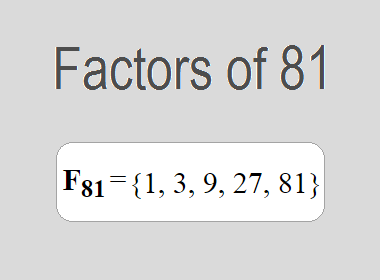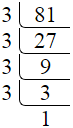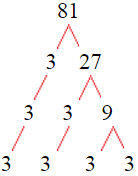# Factors of 81The factors of 81 are 1, 3, 9, 27, and 81 i.e. F81 = {1, 3, 9, 27, 81}. The factors of 81 are all the numbers that can divide 81 without leaving a remainder.

We can check if these numbers are factors of 81 by dividing 81 by each of them. If the result is a whole number, then the number is a factor of 81. Let's do this for each of the numbers listed above:

·        1 is a factor of 81 because 81 divided by 1 is 81.

·        3 is a factor of 81 because 81 divided by 3 is 27.

·        9 is a factor of 81 because 81 divided by 9 is 9.

·        27 is a factor of 81 because 81 divided by 27 is 3.

·        81 is a factor of 81 because 81 divided by 81 is 1.

## How to Find Factors of 81?

1 and the number itself are the factors of every number. So, 1 and 81 are two factors of 81. To find the other factors of 81, we can start by dividing 81 by the numbers between 1 and 81. If we divide 81 by 2, we get a remainder of 1. Therefore, 2 is not a factor of 81. If we divide 81 by 3, we get a remainder of 0. Therefore, 3 is a factor of 81.

Next, we can check if 4 is a factor of 81. If we divide 81 by 4, we get a remainder of 1. Therefore, 4 is not a factor of 81. We can continue this process for all the possible factors of 81.

Through this process, we can find that the factors of 81 are 1, 3, 9, 27, and 81. These are the only numbers that can divide 81 without leaving a remainder.

********************

********************

## Properties of the Factors of 81

The factors of 81 have some interesting properties. One of the properties is that the sum of the factors of 81 is equal to 121. We can see this by adding all the factors of 81 together:

1 + 3 + 9 + 27 + 81 = 121

Another property of the factors of 81 is that they are all odd numbers. This is because 81 is an odd number, and any even number cannot divide an odd number without leaving a remainder.

Another property of the factors of 81 is that the only prime factor of 81 is 3.

## Applications of the Factors of 81

The factors of 81 have several applications in mathematics. One of the applications is in finding the highest common factor (HCF) of two or more numbers. The HCF is the largest factor that two or more numbers have in common. For example, to find the HCF of 81 and 90, we need to find the factors of both numbers and identify the largest factor they have in common. The factors of 81 are 1, 3, 9, 27, and 81. The factors of 90 are 1, 2, 3, 5, 6, 9, 10, 15, 18, 30, 45, and 90. The largest factor that they have in common is 9. Therefore, the HCF of 81 and 90 is 9.

Another application of the factors of 81 is in prime factorization. Prime factorization is the process of expressing a number as the product of its prime factors. The only prime factor of 81 is 3. We can express 81 as:

81 = 3 × 3 × 3 × 3

We can do prime factorization by division and factor tree method also. Here is the prime factorization of 81 by division method,81 = 3 × 3 × 3 × 3

Here is the prime factorization of 81 by the factor tree method,81 = 3 × 3 × 3 × 3## Conclusion

The factors of 81 are the numbers that can divide 81 without leaving a remainder. The factors of 81 are 1, 3, 9, 27, and 81. The factors of 81 have some interesting properties, such as being odd numbers and having a sum of 121. The factors of 81 have several applications in mathematics, such as finding the highest common factor and prime factorization.